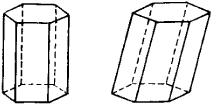# prism

(redirected from Prism (disambiguation))
Also found in: Dictionary, Thesaurus, Medical, Wikipedia.

## prism,

in optics, a piece of translucent glass or crystal used to form a spectrumspectrum,
arrangement or display of light or other form of radiation separated according to wavelength, frequency, energy, or some other property. Beams of charged particles can be separated into a spectrum according to mass in a mass spectrometer (see mass spectrograph).
of light separated according to colors. Its cross section is usually triangular. The light becomes separated because different wavelengths or frequencies are refracted (bent) by different amounts as they enter the prism obliquely and again as they leave it (see refractionrefraction,
in physics, deflection of a wave on passing obliquely from one transparent medium into a second medium in which its speed is different, as the passage of a light ray from air into glass.
). The shorter wavelengths, toward the blue or violet end of the spectrum, are refracted by the greatest amount; the longer wavelengths, toward the red end, are refracted the least. The Nicol prismNicol prism
, optical device invented (1828) by William Nicol of Edinburgh. It consists essentially of a crystal of calcite, or Iceland spar, that is cut at an angle into two equal pieces and joined together again with Canada balsam.
is a special type of prism made of calcite; it is used for polarization of lightpolarization of light,
orientation of the vibration pattern of light waves in a singular plane. Characteristics of Polarization

Polarization is a phenomenon peculiar to transverse waves, i.e.
.

## Prism

A geometric solid with regular polygons at its ends and parallelograms on its sides connecting the ends.

## Prism

a polyhedron in which two of the faces are n-gons (the bases of the prism) and the remaining n faces (the lateral faces) are parallelograms. The bases of a prism are congruent and lie in parallel planes.Figure 1

A prism is called a right prism if the lateral edges are perpendicular to the bases. A right prism is regular if each base is a regular polygon. Prisms can be triangular, quadrangular, and so on, depending on whether the bases are triangles, quadrilaterals, and so on. Figure 1 illustrates a hexagonal prism (the one on the left is also a right prism). The volume of a prism is equal to the product of the base and the altitude (the distance between the bases). See also.

## prism

[′priz·əm]
(crystallography)
A crystal which has three, four, six, eight, or twelve faces, with the face intersection edges parallel, and which is open only at the two ends of the axis parallel to the intersection edges.
(geology)
A long, narrow, wedge-shaped sedimentary body with a width-thickness ratio greater than 5 to 1 but less than 50 to 1.
(mathematics)
A polyhedron with two parallel, congruent faces and all other faces parallelograms.
(optics)
An optical system consisting of two or more usually plane surfaces of a transparent solid or embedded liquid at an angle with each other. Also known as optical prism.

## prism

1. a transparent polygonal solid, often having triangular ends and rectangular sides, for dispersing light into a spectrum or for reflecting and deviating light. They are used in spectroscopes, binoculars, periscopes, etc.
2. a form of crystal with faces parallel to the vertical axis
3. Maths a polyhedron having parallel, polygonal, and congruent bases and sides that are parallelograms

## PRISM

A distributed logic language.

["PRISM: A Parallel Inference System for Problem Solving", S. Kasif et al, Proc 1983 Logic Prog Workshop, pp. 123-152].

## PRISM

(1) (PhotoRefractive Information Storage Materials Consortium) A collaboration of IBM, Stanford University, GTE, Hughes Research Labs, Optitek, SRI International and Rockwell Science Center that is funded by the U.S. government's Advanced Research Projects Agency for the purpose of researching holographic storage.

(2) (PRogrammable Integrated Scripts for Mirror) The programming language for the Mirror communications programs.

(3) See PR/SM.
Site: Follow: Share:
Open / Close